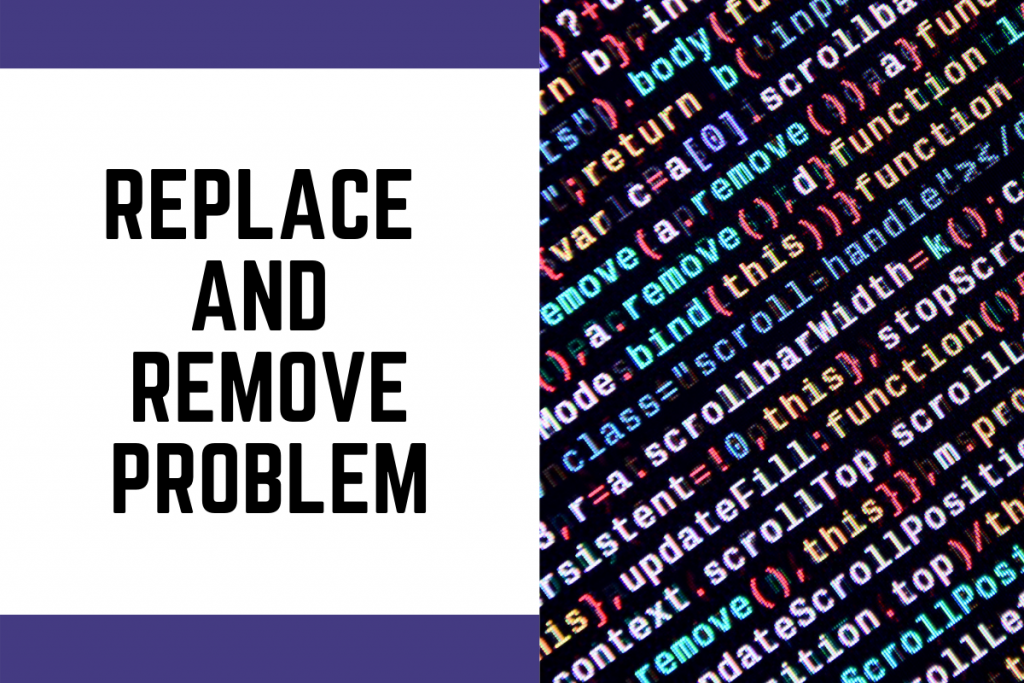# How to Solve the Replace and Remove Problem in Python?Hello coder! So in this tutorial, we will be understanding a simple problem. The name of the problem is `Replace and Remove Problem` where we will be replacing one particular character with a different string and also remove a particular character from the input made by the user.

So, we know that we need to replace one character with a different string or group of characters and remove a character from the input. The two rules that we are gonna follow are as follows:

1. Replace `a` with double d (`dd`)
2. Remove any occurence of `b`

## Solution Implementation

We will be following a number of steps which are mentioned below:

• STEP 1: TAKE INPUT OF ‘N’ ( Input of the initial string )
• STEP 2: CONVERT THE STRING INTO A LIST OF CHARACTER ( Character Array )
• STEP 3: TRAVERSE THROUGH THE CHARACTER ARRAY
• STEP 3.1: IF ‘a’ COMES INTO PICTURE THEN CHANGE IT INTO ‘dd’
• STEP 3.2: IF ‘b’ COMES INTO PICTURE THEN REMOVE IT FROM THE CHARACTER ARRAY
• STEP 4: JOIN THE UPDATED CHARACTER ARRAY INTO THE ORIGINAL STRING AND PRINT THE OUTPUT

Now that we have understood the approach to the problem, let’s move to the implementation part step after another.

### Step 1 and 2: Take input of N and Convert it to character array

Input is taken in Python using the `input` function and then the character array is created using the `list` function which will take the input string as a parameter.

```# 1. Taking input
n = input()
# 2. Convert into a list of characters
l= list(n)
```

### Step 3: Traverse through the array and do replace and remove character following the rules

Now that we have our character array, we will be traversing through the list, and whenever the character `a` is obtained, we replace it with dd, and whenever we encounter `b`, we will remove the character from the character array.

To replace the character we will directly change the character in place in the array and to remove a character from the array we make use of the `remove` function.

```# Rule 1 : Replace 'a' with 'dd'
# Rule 2 : Remove each 'b'

# Iterate over each character
i = len(l)-1
while(i!=-1):

# Rule 1
if(l[i] == 'a'):
l[i] = 'dd'

# Rule 2
elif(l[i] == 'b'):
l.remove(l[i])
i = i-1
```

### Step 4: Join the new updated character array

The last step is to join all the elements of the updated character array into a string. The better option is to change the original string which was taken as an input. The function we use to achieve that is `join` function.

```# Join the updated list
n = ''.join(l)
print("New string is: ",n)
```

And there we go! Your solution is done and complete! Now let’s look at some random sample outputs.

## The Final Code

```# 1. Taking input
n = input()
# 2. Convert into a list of characters
l= list(n)

print("String entered by user is: ",n)

# Rule 1 : Replace 'a' with 'dd'
# Rule 2 : Remove each 'b'

# Iterate over each character
i = len(l)-1
while(i!=-1):

# Rule 1
if(l[i] == 'a'):
l[i] = 'dd'

# Rule 2
elif(l[i] == 'b'):
l.remove(l[i])
i = i-1

# Join the updated list
n = ''.join(l)
print("New string is: ",n)
```

## Outputs

```String entered by user is:  abccba
New string is:  ddccdd
```
```String entered by user is:  abccbabdgsea
New string is:  ddccdddgsedd
```

## Conclusion

I hope you understood the problem and the solution along with code implementation. You can implement everything all by yourself! Thank you for reading! Happy coding!